Question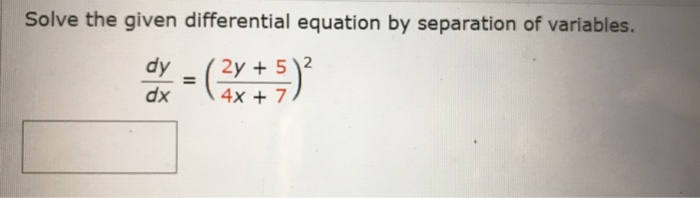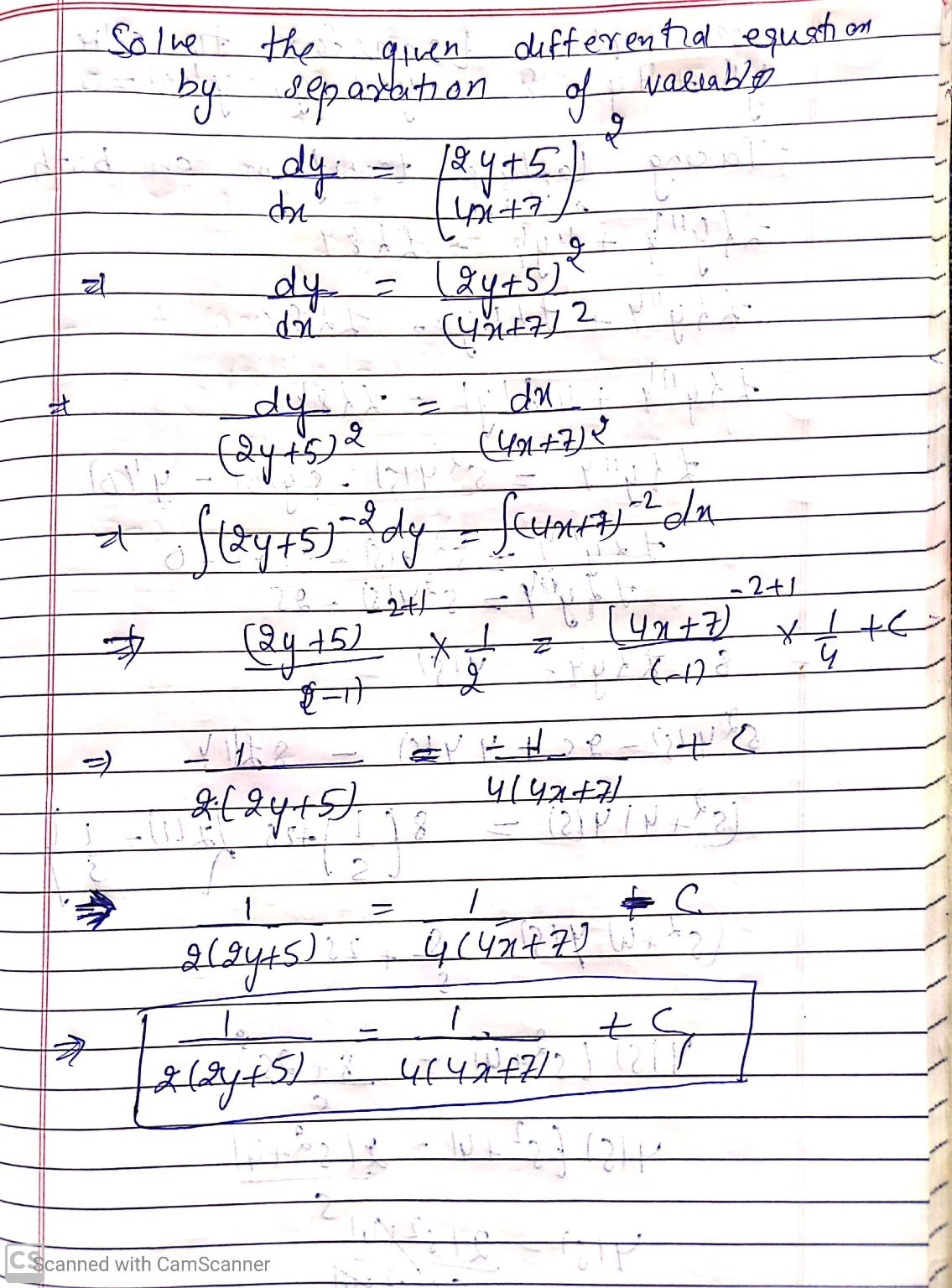#### Earn Coins

Coins can be redeemed for fabulous gifts.

Similar Homework Help Questions
• ### Solve the given differential equation by separation of variables.

Solve the given differential equation by separation of variables. exy(dy/dx) = e-y + e-4x-y

• ### Solve the given differential equation by separation of variables. dy/dx = sin(9x)

Solve the given differential equation by separation of variables. dy/dx =  sin(9x)

• ### Solve the given differential equation by separation of variables.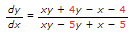Solve the given differential equation by separation of variables. dP/dt= P-P2 Solve the given differential equation by separation of variables. dN/dt + N = Ntet+3 Solve the given differential equation by separation of variables. Find an explicit solution of the given initial-value problem.

• ### Solve the given differential equation by separation of variables.

Solve the given differential equation by separation of variables. (ey + 1)2e-y dx + (ex + 1)8e-x dy = 0

• ### Solve the given differential equation by separation of variables. dy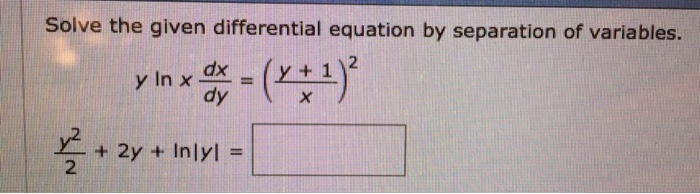Solve the given differential equation by separation of variables. dy

• ### Problem 4. Verify that the differential equation is exact then solve it! (4x + 2y)dx +...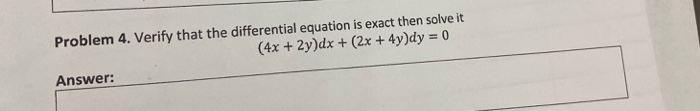Problem 4. Verify that the differential equation is exact then solve it! (4x + 2y)dx + (2x + 4y)dy = 0 Answer:

• ### Solve the given differential equation. (x2 4) dy (2x - 10xy) dx + 1 + 5...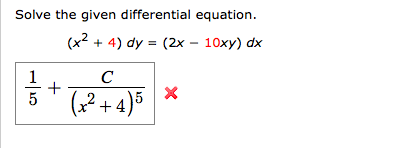Solve the given differential equation. (x2 4) dy (2x - 10xy) dx + 1 + 5 2+4)5 Solve the given differential equation. (x2 4) dy (2x - 10xy) dx + 1 + 5 2+4)5

• ### Solve the following partial differential equation using separation of variables method to determine the function 0...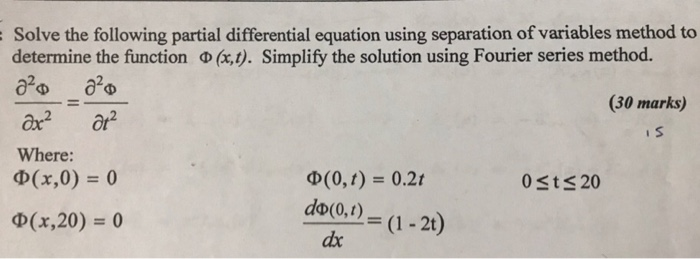Solve the following partial differential equation using separation of variables method to determine the function 0 (x,t). Simplify the solution using Fourier series method. 2²0 2²0 (30 marks) Ox² at is Where: (x,0) = 0 0(0,t) = 0.21 0<t< 20 Q(x,20) = 0 do(0,1)= (1 - 2t) dx = (1-21)

• ### 2. Solve the differential equation (2xy + y)dx + (x2 + 3.ry2 – 2y)dy = 0....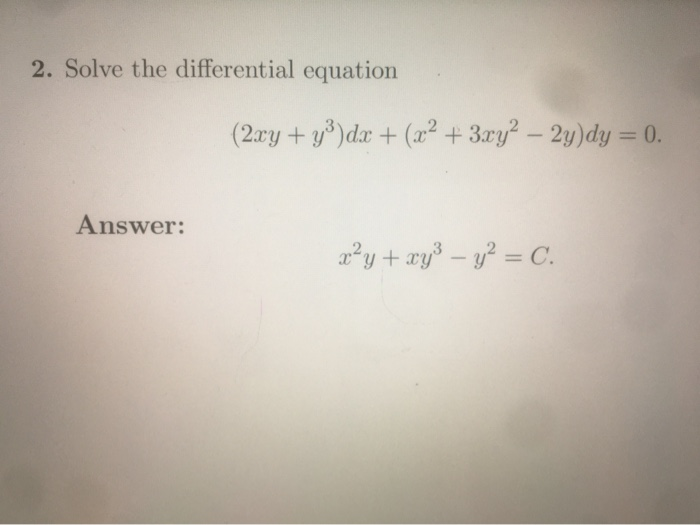2. Solve the differential equation (2xy + y)dx + (x2 + 3.ry2 – 2y)dy = 0. Answer: x²y + xy3 – y2 = C.

• ### of 3. Solve the differential equation y de + - dx +(2y Inx - 3sin (3y))...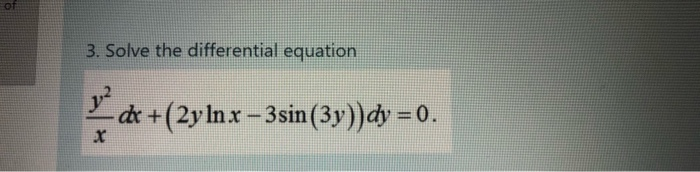of 3. Solve the differential equation y de + - dx +(2y Inx - 3sin (3y)) dy = 0.